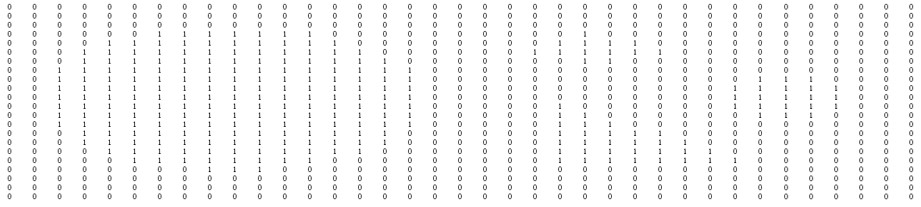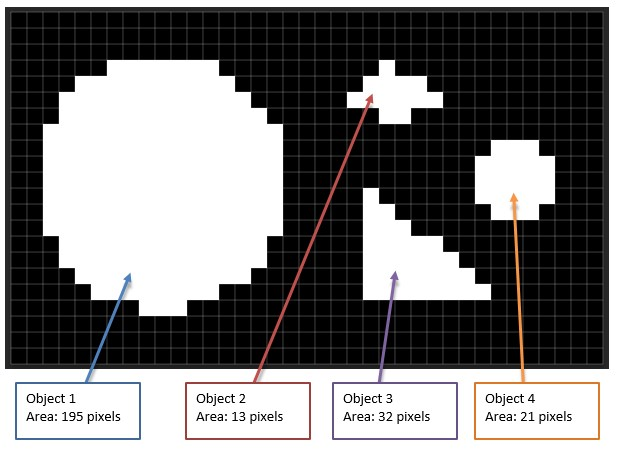This How-to applies to Image Analysis. It shows a method of using Matlab to count objects and find the subsequent area of those objects.

The following image will serve as an example:The image simply has 4 geometric shapes on a black background. The goal is to count the number of objects and to find the area of each of those objects.

For documenting purposes (and so that we can see what the numerical arrays look like), the test image is cropped and scaled down to a small size of 37×22 pixels:Here are what the actual values look like:Here is what a binary version of the image looks like as an array. The four simple shapes are indicated by the use of the number one for white, with a background of zeros for black:## Counting and Indexing the Objects with the Function: bwlabel

The Matlab function “bwlabel” is used to both separate, and count the objects. (More info of the “bwlabel” function can be found on the Matworks web site:
http://www.mathworks.com/help/images/ref/bwlabel.html). If the binary image has been assigned to the variable “bmask”, the function is called as follows:

```[indeximgarray,count] = bwlabel(bmask); ```

For this example, the result for count will be 4 and the index array would look as follows.
``` >> count 4 ```Note that each object now has a unique integer value.

## Separating the Objects

Next the index array is separated. In the following “for” loop, each object is assigned to a different z value of a three dimensional array (note from the preceding step, the variable “count” has the value of 4).:
``` for i=1:count objseparate(:,:,i) = indeximgarray == i; end ```
(Having a mask file, for each object, located on separate array layers, is handy for various analysis techniques.)

## Finding the Area

The next step is to find the area; and a very simple and straight forward method is to simply sum up the values in the array that contains the separate objects. This works since each element has a value of either “1”, if the object exists at that position, or “0” if the element position is part of the background.
``` objarea2 = sum(sum(objseparate));```

(The function sum addes up the values in the columns, so we use sum twice. More info on the sum functions is available at the Mathworks web site: http://www.mathworks.com/help/matlab/ref/sum.html)

And the results of the sum function:
``` >> objarea2 objarea2(:,:,1) = 195 objarea2(:,:,2) = 13 objarea2(:,:,3) = 32 objarea2(:,:,4) = 21 ```

## Illustration of Results: